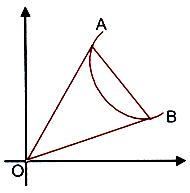×#### Thank you for registering.

One of our academic counsellors will contact you within 1 working day.

Click to Chat

1800-1023-196

+91-120-4616500

CART 0

• 0

MY CART (5)

Use Coupon: CART20 and get 20% off on all online Study Material

ITEM
DETAILS
MRP
DISCOUNT
FINAL PRICE
Total Price: Rs.

There are no items in this cart.
Continue Shopping```Joint Equation of Pair of Lines

Joint Equation of Pair of Lines Joining the Origin and the Points of Intersection of a Line and a CurveIf the line lx + my + n = 0, (n ≠ 0) i.e. the line not passing through origin) cuts the curve ax2 + by2 + 2gx + 2fy + c = 0 at two points A and B, then the joint equation of straight lines passing through A and B and the origin is given by homogenizing the equation of the curve by the equation of the line. i.e.ax2 + 2hxy + by2 + (2gx + 2fy) (kx+my/–n) + c (lx+my/–n)2 = 0.

is the equation of the lines OA and OB.

Illustration:

Prove that the straight lines joining the origin to the points of intersection of the straight line hx + ky = 2hk and the curve (x – k)2 + (y – y)2 = c2 are at right angles if h2 + k2 = c2.

Solution:

Making the equation of the curve homogeneous with the help of that of the line, we get

x2 + y2 –2(kx + hy) (hx+ky/2hk) + (h2 + k2 – c2) (hx+ky/2hk) = 0

or 4h2k2x2 + 4h2k2y2 – 4h2x(hx+ky) – 4h2ky(hx + ky) + (h2 + k2 – c)(h2x2 + k2y2 + 2hxy) = 0.

This is the equation of the pair of lines joining the origin to the points of intersection of the given line and the curve. They will be at right angles if

Coefficient of x2 + coefficient of y2 = 0 i.e.

(h2 + k2) (h2 + k2 – c2) = 0 ⇒ h2 + k2 = c2  (since h2 + k2 ≠ 0).

The platform at askIITians is the only group of IITians which provides you free online courses and a good professional advice for IIT JEE, AIEEE and other Engineering Examination preparation. You can also visit askIITians.com to study topics pertaining to the IIT JEE and AIEEE syllabus for free.

To read more, Buy study materials of Straight Lines comprising study notes, revision notes, video lectures, previous year solved questions etc. Also browse for more study materials on Mathematics here.

To know more about the study material of engineering and medical exams, please fill up the form given below:

```### Course Features

• 731 Video Lectures
• Revision Notes
• Previous Year Papers
• Mind Map
• Study Planner
• NCERT Solutions
• Discussion Forum
• Test paper with Video Solution